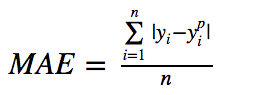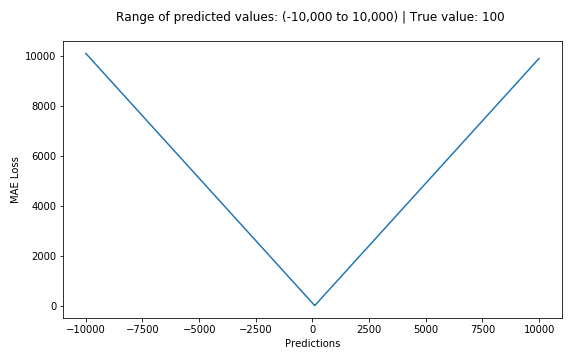# ML

ML

Few terms to begin with:-

Loss Function: tells how good a prediction model is in terms of how close it is able to predict the expected outcome.

Objective Function: algorithms rely on maximizing and minimizing a function called objective fn, and the group of functions that are minimized are known as loss function.

Gradient Descent: if a mountain with smooth slopes is loss function, then GD is sliding down that smooth mountain to reach the bottom-most point. It is the most common way to find the min. point of a function.

Categorization of Loss functions: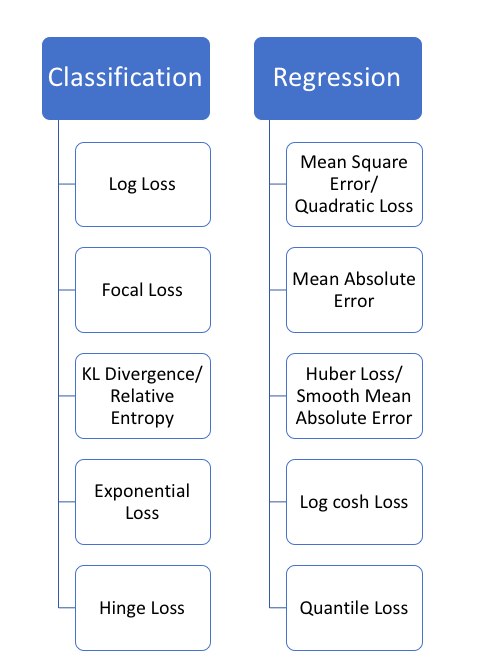No need to remember all this at the moment. Just know that:

predicts a label                                         |            predicts a quantity

Regression Loss:

-Most commonly used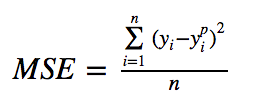-sum of sq. distances of target variable and predicted variable

— aka Mean Square Error (or Quadratic Loss, L2 Loss)

Further graphs are going to use the example, where:

• target value is 100
• predicted values range between -10,000 to 10,000
• The MSE loss becomes minimum when actual value is 100 (i.e. predicted value^2-actual value^2 = 0)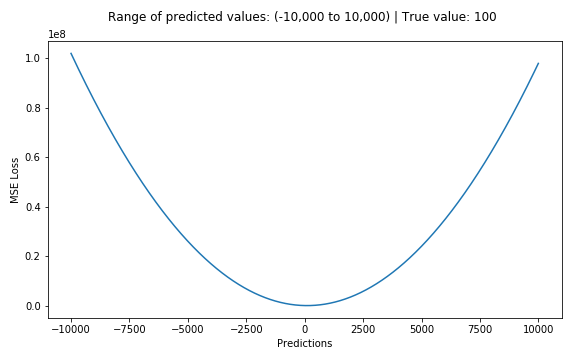– Yet another loss function is where:

• the sum of absolute differences between our target and predicted variables
• it measures average magnitude of errors in a set of predictions
— aka Mean Absolute Error